Open in App
Not now

# Surface Area of a Pyramid Formula

• Last Updated : 14 Jul, 2022

Pyramid is a three-dimensional geometric structure with a polygonal base and all its triangular faces meet at a common point called the apex or vertex. The real-life examples of pyramids are the pyramids of Egypt; rooftops; camping tents, etc. Depending upon the shape of the polygonal base, pyramids are classified into different types, such as triangular pyramids, square pyramids, rectangular pyramids, etc. The side faces, or lateral surfaces of a pyramid, are triangles that meet at a common point called the apex. The height, or altitude, of a pyramid, is the perpendicular distance between the apex and the center of the base, whereas a slant height is a perpendicular distance between the apex and the base of a lateral surface.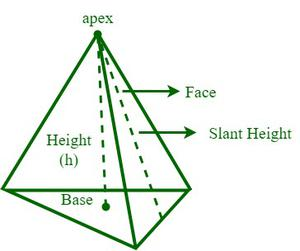### Surface Area of a Pyramid

The surface area of a pyramid is the sum of the areas of the lateral surfaces, or side faces, and the base of a pyramid. The surface area of a pyramid has basically two types of surface areas: the lateral surface area and the total surface area, and they are measured in terms of m2, cm2, in2, ft2, etc.

Lateral surface area of a pyramid (LSA) = Sum of areas of the lateral surfaces (triangles) of the pyramid

Total surface area of a pyramid (TSA) = Lateral surface area of the pyramid + Area of the base

To determine the lateral surface of a pyramid, the areas of the side faces (triangles) are calculated and the results are added, whereas the total surface area of a pyramid is calculated by adding the area of the pyramid’s base and the lateral surface area.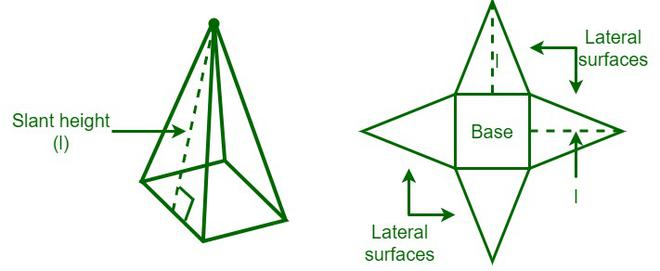Surface area of a Triangular pyramid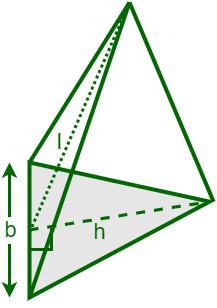A triangular pyramid is a pyramid having a triangular base, where the triangular base can be equilateral, isosceles, or a scalar triangle. It has three lateral (triangular) faces and a triangular base.

We know that,

The total surface area of a pyramid (TSA) = Lateral surface area of the pyramid + Area of the base

Lateral surface area (LSA) = ½ × perimeter × slant height

So, TSA = ½ × perimeter × slant height + ½ × base × height

Total surface area (TSA) of a triangular pyramid = ½ ×  P ×  l + ½ bh

where,

P is perimeter of base

l is slant height of pyramid

b is base of base triangle

h is height of pyramid

Surface area of a Square pyramid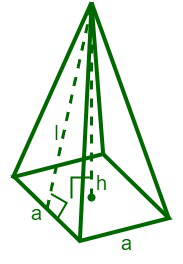A square pyramid is a pyramid having a square base. It has four lateral (triangular) faces and a square base.

We know that,

The total surface area of a pyramid (TSA) = Lateral surface area of the pyramid + Area of the base

The slant height of the pyramid (l) = √[(a/2)2 + h2]

LSA = 4 × [½ × a × l] = 2al

Lateral surface area of the square pyramid (LSA)= 2al

So, TSA = 2al + a2

Total surface area of a square pyramid (TSA) = 2al + a2

where,

a is side of square base

l is slant height of pyramid

Surface area of a rectangular pyramid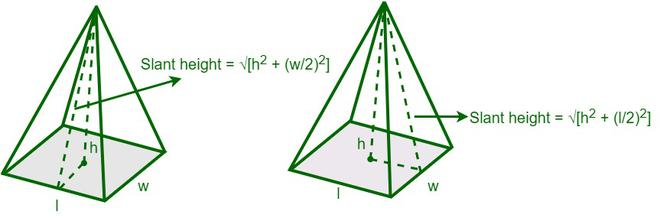A rectangular pyramid is a pyramid having a rectangular base. It has four lateral (triangular) faces and a rectangular base.

We know that,

The total surface area of a pyramid (TSA) = Lateral surface area of the pyramid + Area of the base

The slant height of length face of the pyramid = √[h2 + (l/2)2]

The slant height of width face of the pyramid = √[h2 + (w/2)2]

Lateral surface area of a rectangular pyramid = 2 × {½ × l ×√[h2 + (l/2)2]} + 2 × {½ × w ×√[h2 + (w/2)2]

So,

Total surface area of the rectangular pyramid = 2 × {½ × l ×√[h2 + (l/2)2]} + 2 × {½ × w ×√[h2 + (w/2)2] + l × w

where,

l is length of rectangle base

w is breadth of rectangle base

h is height of pyramid

Surface area of a pentagonal pyramid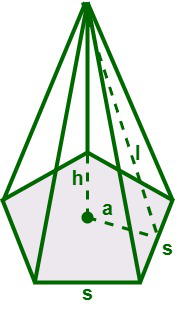A pentagonal pyramid is a pyramid having a pentagonal base. It has five lateral (triangular) faces and a pentagonal base.

We know that,

The total surface area of a pyramid (TSA) = Lateral surface area of the pyramid + Area of the base

The apothem length of the base = a

The side length of the base = s

The slant height of the pyramid = l

The area of the pentagonal base = 5⁄2 (a × s)

Now,

LSA = 5 × [½ × base × height] = 5/2 × s × l

Lateral surface area of the pentagonal pyramid = 5⁄2 (s × l)

Total surface area of the pentagonal pyramid = 5⁄2 (s × l) + 5⁄2 (a × s)

where,

l is  slant height of the pyramid

a apothem length of the base

s side length of the base

Surface area of a hexagonal pyramid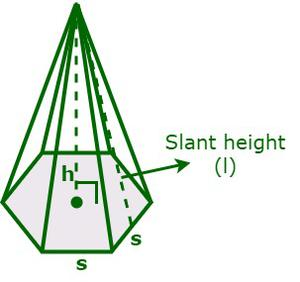A hexagonal pyramid is a pyramid having a hexagonal base. It has six lateral (triangular) faces and a hexagonal base.

We know that,

The total surface area of a pyramid (TSA) = Lateral surface area of the pyramid + Area of the base

The side length of the base = s

The slant height of the pyramid = l

Area of the hexagonal base = 3√3/2 (s)2

Now,

LSA = The sum of areas of the lateral surfaces (triangles) of the pyramid

= 6 × [½ × base × height] =3(s × l)

Lateral surface area of the hexagonal pyramid = 3(s × l)

Total surface area of the hexagonal pyramid = 3(s × l) + 3√3/2 (s)2

where,

s is side length of the base

l is slant height of the pyramid

### Sample Problems

Problem 1: Determine the surface area of a square pyramid if the side length of the base is 16 inches and the slant height of the pyramid is 18 inches.

Solution:

Given,

The side of the square base (a) = 16 inches, and

Slant height, l = 18 inches

The perimeter of the square base (P) = 4a = 4(16) = 64 inches

The lateral surface area of a square pyramid = (½) Pl

LSA = (½ ) × (64) × 18 = 576 sq. in

Now, the total surface area = Area of the base + LSA

= a2 + LSA

= (16)2 + 576 = 832 sq. in

Hence, the surface area of the given pyramid is 832 sq. in.

Problem 2: A box is in the shape of a triangular pyramid, and every face of the box is in the shape of an equilateral triangle. Now, determine the total surface area of a triangular pyramid if the side length of the base is 24 cm.

Solution:

Given data,

The side of the base (a) = 24 inches, and

We know that,

The total surface area of a pyramid (TSA) = Area of the base + Area of lateral faces

We have,

Area of an equilateral triangle = √3/4 (a)2

Hence, the area of each triangular face = √3/4 (a)2

= √3/4 (24)2

= 249.415 sq. cm

Now, TSA = 249.415 + 3 × (249.415) = 997.66 sq. cm

Hence, the total surface area of the given pyramid is 997.66 sq. cm.

Problem 3: Determine the lateral surface area of a pentagonal pyramid if the side length of the base is 10 cm and the slant height of the pyramid is 15 inches.

Solution:

Given,

The side of the pentagonal base (a) = 10 inches, and

Slant height, l = 15 inches

The perimeter of the square base (P) = 5a = 5(10) = 50 cm

The lateral surface area of a pentagonal pyramid is,

Lateral surface area (LSA) = (½) Pl

= (½ ) × (50) × 15

= 375 sq. cm

Hence, the lateral surface area of the given pyramid is 375 sq. cm.

Problem 4: Determine the surface area of a hexagonal pyramid if the side length of the base is 12 inches and the slant height of the pyramid is 14 inches.

Solution:

Given,

The side of the hexagonal base (a) = 12 inches, and

Slant height, l = 14 inches

The perimeter of the square base (P) = 6a = 6(12) = 72 inches

The surface area of a pentagonal pyramid = Area of the base + Area of lateral faces

Lateral surface area (LSA) = (½) Pl

= (½ ) × (72) × 14 = 504 sq. in

Area of the hexagonal base = 3√3/2 (a)2 = 3√3/2 (12) = 374.123 sq. in

The surface area of a pyramid = Area of the base + Area of lateral faces

= 374.123 sq. in + 504 sq. in = 878.123 sq. in

Hence, the surface area of the given pyramid is 878.123 sq. in.

Problem 5: Determine the side length of a square pyramid if its lateral surface area is 600 square inches and the slant height of the pyramid is 20 inches.

Solution:

Given,

The  lateral surface area = 600 square inches

Slant height, l = 20 inches

Let the side length of a square base be “a”.

Now, the perimeter of the base (P)= 4a

We know that

The lateral surface area of a square pyramid = (½) Pl

⇒ 600 = (½ ) × (4a) × 20

⇒ 80a = 1200 ⇒ a = 15 inches

Hence, the side length of the given pyramid is 15 in.

My Personal Notes arrow_drop_up
Related Articles